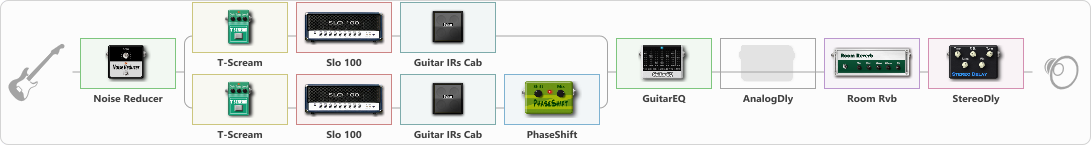# 123

Discussion in 'ToneLib-GFX presets' started by Dabir, Aug 6, 2022.

1. 123

Preset name: Progressive vibe

Effects chain:Effect: "Noise Reducer" (Dynamics / Filter), active - "yes"
{
"Sens" = 82
"Mode" = Hard
}

Effect: "Splitter" (Dynamics / Filter)
{
"A-Bypass" = Off
"A-Pan" = -55
"A-Level" = 60
"B-Bypass" = Off
"B-Pan" = 62
"B-Level" = 55
"Width" = 0

'A' branch:
{

Effect: "T-Scream" (Overdrive / Distortion), active - "yes"
{
"Drive" = 0
"Tone" = 77
"Level" = 100
}

Effect: "Slo 100" (Amp simulators), active - "yes"
{
"Gain" = 87
"Bass" = 66
"Middle" = 65
"Treble" = 44
"Presence" = 42
"Master" = 62
"Level (dB)" = 0
}

Effect: "Guitar IRs Cab" (Cabinets), active - "yes"
{
"Model" = Mesa Rectifier (4x12")
"Mic Position" = Middle
"Mic Distance" = Middle
"Low Cut (Hz)" = 60
"Hi Cut (kHz)" = 20.0
"Mix" = 100
"Level (dB)" = 2
}
}
'B' branch:
{

Effect: "T-Scream" (Overdrive / Distortion), active - "yes"
{
"Drive" = 0
"Tone" = 77
"Level" = 100
}

Effect: "Slo 100" (Amp simulators), active - "yes"
{
"Gain" = 87
"Bass" = 66
"Middle" = 65
"Treble" = 44
"Presence" = 42
"Master" = 62
"Level (dB)" = 0
}

Effect: "Guitar IRs Cab" (Cabinets), active - "yes"
{
"Model" = Marshall JCM2000 (4x12")
"Mic Position" = Edge
"Mic Distance" = Far
"Low Cut (Hz)" = 60
"Hi Cut (kHz)" = 20.0
"Mix" = 100
"Level (dB)" = 2
}

Effect: "PhaseShift" (Modulation / Sfx), active - "yes"
{
"Shift" = 75
"Mix" = 100
}
}
}

Effect: "GuitarEQ" (Dynamics / Filter), active - "yes"
{
"160 Hz" = -8
"400 Hz" = -7
"800 Hz" = 0
"1.6 kHz" = 0
"3.2 kHz" = 0
"6.4 kHz" = -4
"12 kHz" = -6
"Level (dB)" = 2
}

Effect: "AnalogDly" (Delay), active - "no"
{
"Time" = 514
"Feedback" = 49
"Tone" = 42
"Mix" = 65
}

Effect: "Room Rvb" (Reverberation), active - "yes"
{
"Time" = 6.2
"PreDelay" = 0
"LoDamp" = 100
"HiDamp" = 25
"Mix" = 49
}

Effect: "StereoDly" (Delay), active - "yes"
{
"Time" = 228
"Feedback" = 36
"Tone" = 56
"Sens" = 35
"Mix" = 36
}

Note: You will need to download and install the ToneLib-GFX software to use the preset.

File size:
1.2 KB
Views:
2,032